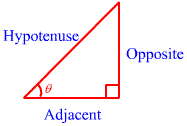## Show basic trigonometric functions, Mathematics

Assignment Help:

Q. Show basic Trigonometric Functions?

Ans.

There are six trigonometric functions and they can be defined using a right angle triangle. We first label each side according to the angle we are interested in. Consider the angle in the diagram. The horizontal side is adjacent to the angle, so we label this side adjacent. The vertical line is opposite from the angle , so we label it opposite. The hypotenuse is always the side across from the right angle.The three most important trigonometric functions are sine, cosine and tangent. They can be defined with abbreviations as follows:Since these are extremely important functions, it would be very helpful if you learn these ratios. You may be able to remember them better if you consider the ‘word' SOH CAH TOA.

#### One-sided limits, One-sided limits: We do this along with one-sided limits...

One-sided limits: We do this along with one-sided limits.  As the name implies, with one-sided limits we will just looking at one side of the point in question.  Following are the

#### Topological spease, let X be a nonempty set. let x belong to X. show that t...

let X be a nonempty set. let x belong to X. show that the collection l={ union subset of X : union = empty or belong U

#### Detremine the surface area to the nearest inch, If a tabletop has a diamete...

If a tabletop has a diameter of 42 in, Detremine the surface area to the nearest inch? (π = 3.14) a. 1,384 in 2 b. 1,319 in 2 c. 1,385 in 2 d. 5,539 in 2 c. Th

#### Division, 50387 divided by 21

50387 divided by 21

Doing the following exercise will give you and opportunity to think about this aspect of children. E1) List some illustrations of exploration by four or five-year-olds that you

#### Find the surface area and volume of the composite figure, SA= Ph+2B L=36 ft...

SA= Ph+2B L=36 ft W=10 ft H=20 ft P=92 ft B=360 ft

#### What is box-and-whisker plot, Q. What is Box-and-Whisker Plot? Ans. ...

Q. What is Box-and-Whisker Plot? Ans. Line graphs or stem-and-leaf plots become difficult to manage when there is a large amount of data. Box-and-whisker plots help summa

#### Domain and range of a function , Domain and range of a functio:  One of th...

Domain and range of a functio:  One of the more significant ideas regarding functions is that of the domain and range of a function. In simplest world the domain of function is th

#### Developing an understanidng of multiplication, DEVELOPING AN UNDERSTANIDNG ...

DEVELOPING AN UNDERSTANIDNG OF MULTIPLICATION :  The most important aspect of knowing multiplication is to understand what it means and where it is applied. It needs to be first i

#### Ann, What was last years salary if after a 3% increase the salary is 35,020...

What was last years salary if after a 3% increase the salary is 35,020?